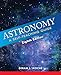# Why we know the number of stars in the universe is finite

## A simple proof to show the number of stars in the universe is finite

How many stars are there in the universe? Nobody knows the answer to that, but – as Steve Rogerson explains here - we can prove mathematically that the number of stars must be finite rather than infinite as some claim. And the basis of this proof is standing outside and looking up into the night sky.A finite number of stars in an infinite universePhoto from NASA

Some people have argued that because the universe is infinite, therefore the number of stars within the universe must also be infinite. But a simple look up into the night sky (or the day sky come to that) offers a simple proof that the number of stars must be finite.

If there was an infinite number of stars in the universe, you would be blinded every time you looked up into the sky. Quite simply, an infinite number of stars would produce an infinite amount of light.

But not all the light reaches the Earth

Ah, but surely a lot of the light is lost on the way, absorbed into other bodies, deflected, reflected and so on. Thus not all the light that is created reaches the Earth.

True, not all the light reaches the Earth but some of it does, and that is the key point of the proof. If no light from the stars reached the Earth, it could be argued that the number of stars was infinite and something was stopping that light getting here.

However, some light from the stars does the reach the Earth. By definition, that must be a percentage of the total light that is created. Now, part of the basic maths of infinity states that any percentage of infinity is in itself infinity. So, 50% of infinity is also infinity, as is 0.5%, or even 0.000000005%. No matter how small the proportion, it must still be infinity.

Now, the amount of light that does reach the Earth from the stars has to be a proportion of the light that is created, even if it is a very small proportion. If there were an infinite number of stars thus creating an infinite amount of light it would therefore follow that the percentage of that light reaching Earth would also be infinite. But we know that the amount of light reaching Earth is finite – because we are not blinded when we look up in the sky – therefore the total amount of light created by the stars must also be finite. Therefore, the number of stars in the universe is also finite.Astronomy: A Self-Teaching Guide, Eighth Edition (Wiley Self Teaching Guides)For a generation, Astronomy: A Self-Teaching Guide has introduced hundreds of thousands of readers worldwide to the night sky. Now this classic beginner's guide has been complet...View on Amazon3D Crystal Ball with Solar System model and LED lamp Base, Clear 80mm (3.15 inch) Solar System Cr...Note: This crystal ball cannot rotate itself, it's still, please kindly noted it.Product features: 1. This crystal ball of solar system displays 8 planets around the Sun ( not i...View on Amazon
Updated: 05/29/2016, SteveRogerson
2

Only logged-in users are allowed to comment. Login

#### You might also like

Why do buses bunch?

You wait half an hour for a bus and then three come along at once. But why?

All About the Big Bang Theory

The universe got created according to the principles of Big Bang theory. Like...

Disclosure: This page generates income for authors based on affiliate relationships with our partners, including Amazon, Google and others.